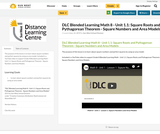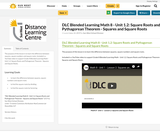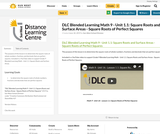# 3 Results

View
Selected filters:
• Perfect SquareConditional Remix & Share Permitted
CC BY-NC-SA
Rating

The purpose of this lesson is to learn about square numbers and perfect squares by using an area model.

Included is a YouTube video to support Grade 8 Blended Learning Math - Unit 1.1: Square Roots and Pythagorean Theorem - Square Numbers and Area Models.

Subject:
Math
Material Type:
Activity/Lab
Homework/Assignment
Lesson
Provider:
Sun West Distance Learning Centre (DLC)
Author:
Sun West School Division
06/14/2019Conditional Remix & Share Permitted
CC BY-NC-SA
Rating

The purpose of this lesson is to learn the difference between squares, square numbers and square roots.

Included is a YouTube video to support Grade 8 Blended Learning Math - Unit 1.2: Square Roots and Pythagorean Theorem - Squares and Square Roots.

Subject:
Math
Material Type:
Activity/Lab
Homework/Assignment
Lesson
Provider:
Sun West Distance Learning Centre (DLC)
Author:
Sun West School Division
06/14/2019Conditional Remix & Share Permitted
CC BY-NC-SA
Rating

The purpose of this lesson is to determine the square roots of whole numbers, fractions and decimals that are perfect squares.

Included is a YouTube video to support Grade 9 Blended Learning Math - Unit 1.1: Square Roots and Surface Areas.

Subject:
Math
Material Type:
Activity/Lab
Homework/Assignment
Lesson
Provider:
Sun West Distance Learning Centre (DLC)
Author:
Sun West School Division